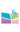﻿2019.11.29，康静教授，西北大学，Higher-dimensional Dispersive Quantization-中国矿业大学（北京）理学院网站2019.11.29，康静教授，西北大学，Higher-dimensional Dispersive Quantization

 题  目： Higher-dimensional Dispersive Quantization 报告人： 康静 教授，西北大学 摘  要： In this talk, we will investigate the dispersive quantization of the two-dimensional linear dispersive equations subject to the periodic initial-boundary value problem over a bounded domain on the plane. We first study the periodic initial-boundary value problem for the two-dimensional linear KdV equation on a rectangle domain. We show that the piecewise constant initial data leads to quantized structures at rational times, meaning that the solution is piecewise constant on rational sub- rectangle. Furthermore, we verify these results extend to general two-dimensional linear dispersive equations with “rational polynomial'' dispersion relations, subject to a more general piecewise smooth initial condition. The solution is a linear combination of finitely many translates of the initial data. Next, we show that in the case of pseudo-periodic boundary conditions, the solution of the initial-boundary value problem for the two-dimensional Schrödinger equation over a rectangle domain exhibits the phenomenon of revival at rational times. Namely, it is a linear combination of a certain number of copies of the initial data. 时  间： 11月29日 星期五 8:30—10:00 地  点： 逸夫楼1537 邀请人： 田凯 副教授